Question

# Three long straight wires are parallel to each other and carry currents directed into or out...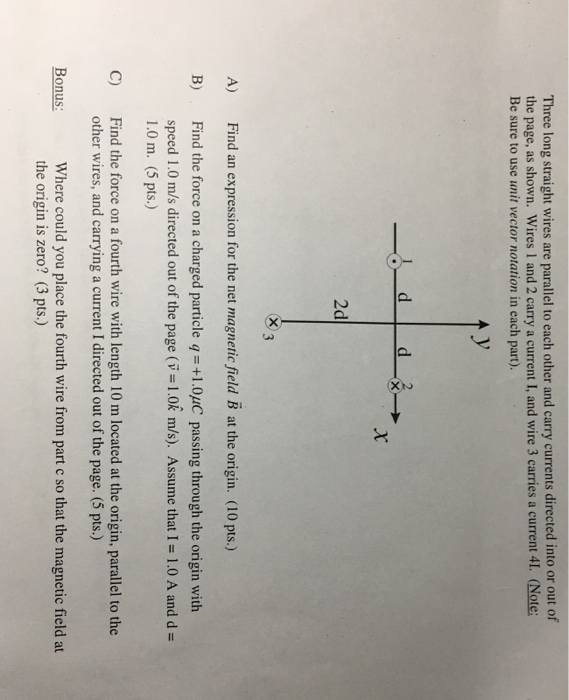Three long straight wires are parallel to each other and carry currents directed into or out of the page, as shown. Wires 1 and 2 carry a current I, and wire 3 carries a current 41. (Note: Be sure to use unit vector notation in each part). 2d indn rssio for th net magnaie fald B ) B) Find the force on a charged particle q-+1.0uC passing through the origin with speed 1.0 m/s directed out of the page (v-1.0k m/s). Assume that I-1.0 A and d- 1.0 m. (5 pts.) Find the force on a fourth wire with length 10 m located at the origin, parallel to the other wires, and carrying a current I directed out of the page. (5 pts.) C) Where could you place the fourth wire from part c so that the magnetic field at the origin is zero? (3 pts.) Bonus: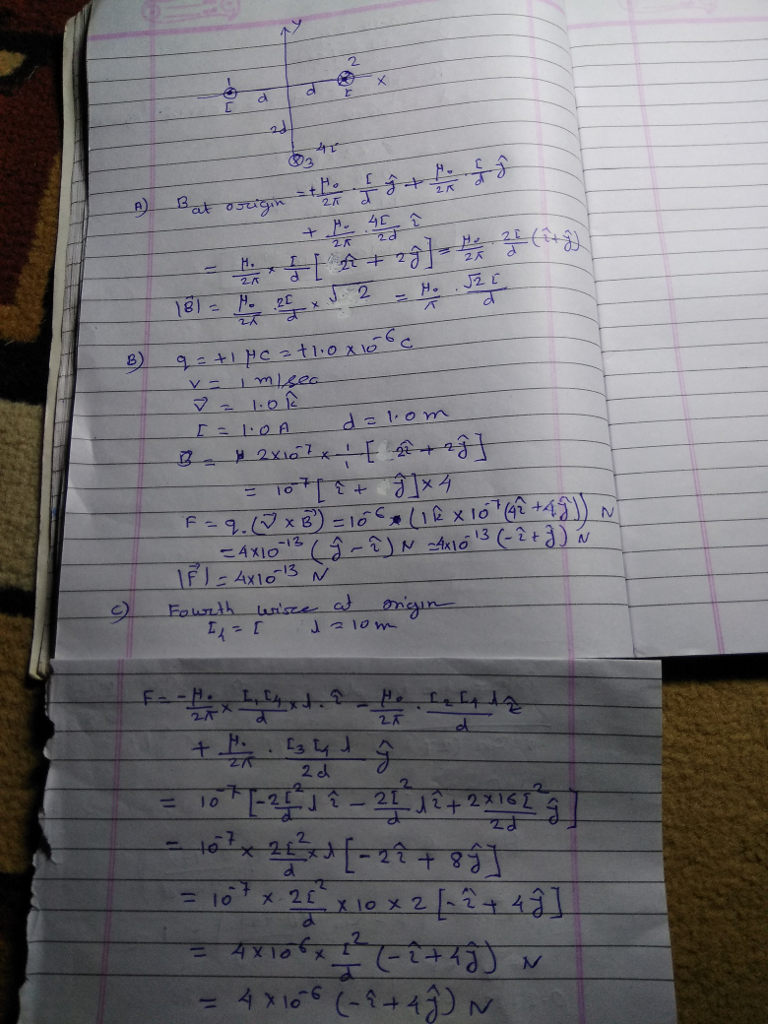#### Earn Coins

Coins can be redeemed for fabulous gifts.

Similar Homework Help Questions
• ### Problem 1 Three long straight wires are parallel to each other and carry currents directed into...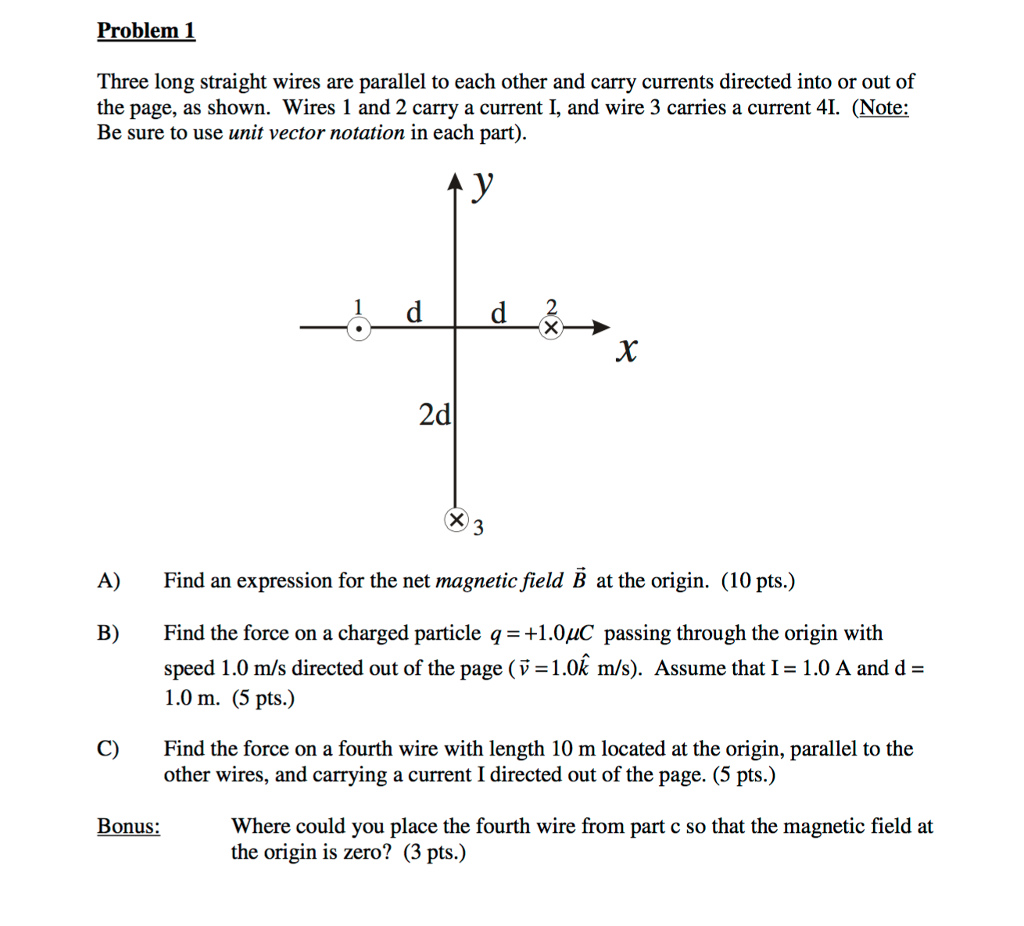Problem 1 Three long straight wires are parallel to each other and carry currents directed into or out of the page, as shown. Wires 1 and 2 carry a current I, and wire 3 carries a current 4. (Note: Be sure to use unit vector notation in each part) 2 2d A) Find an expression for the net magnetic field B at the origin. (10 pts.) B) Find the force on a charged particle q1.0uC passing through the origin with...

• ### Three long wires, all parallel to each other, each carry a current of 10 A out...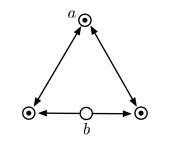Three long wires, all parallel to each other, each carry a current of 10 A out of the page. The wires sit on the vertices of an equilateral triangle with sides of 12.5 cm, as shown below. A fourth wire (wire b) passes through the midpoint of the base of the triangle and is parallel to the other three wires. What is the net magnetic force on wire a (the top vertex of the triangle) if wire b carries a...

• ### Two very long, straight, parallel wires carry currents that are directed perpendicular to the page as...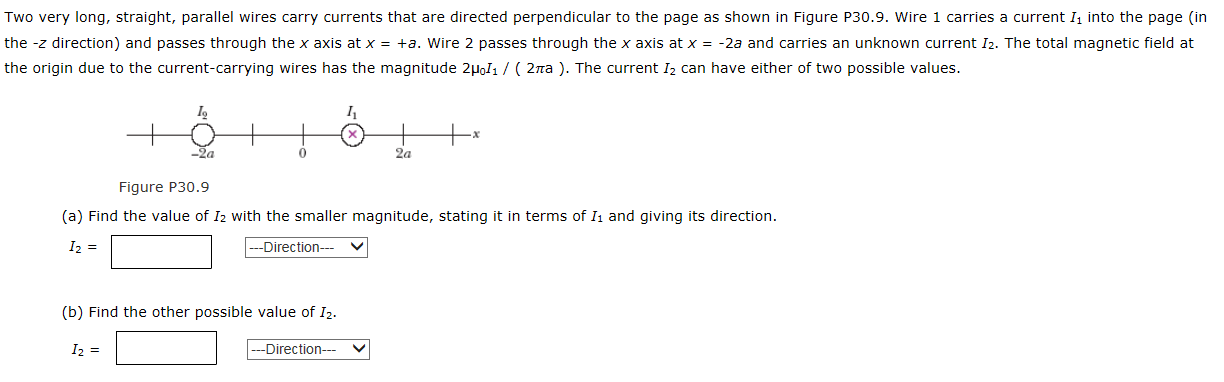Two very long, straight, parallel wires carry currents that are directed perpendicular to the page as shown in Figure P30.9. Wire 1 carries a current 1. into the page (in the -z direction) and passes through the x axis at x = -2a. Wire 2 passes through the x axis at x = -+a and carries an unknown current 12. The total magnetic field at the origin due to the current-carrying wires has the magnitude 2PoIi / ( 2 pira...

• ### Three very long, straight parallel wires each carry currents of I, directed out of the page....Three very long, straight parallel wires each carry currents of I, directed out of the page. The wires pass through the vertices of a right isosceles triangle of side d. What is the magnitude of the magnetic field at a point P at the midpoint of the hypotenuse of the triangle? There is no diagram.

• ### 2 long straight wires run parallel to each other, and carry currentS flowing in the same...

2 long straight wires run parallel to each other, and carry currentS flowing in the same direction. Wire 1 carries a current of 1.2A,and wire 2 carries a current of 1.6A. The wires are 15 cm apart.a) What is the magnetic field from wire 1 at the location of wire 2?b)What is the magnetic force per unit length felt by wire 2? C)What is the magnetic force per unit length felt by wire 1?

• ### Two long straight wires run parallel to each other, and carry currents flowing in the same...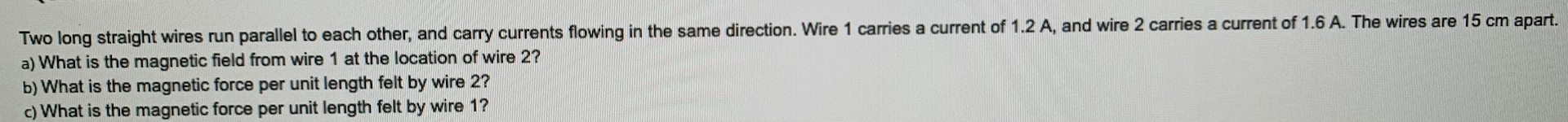Two long straight wires run parallel to each other, and carry currents flowing in the same direction. Wire 1 carries a current of 1.2 A, and wire 2 carries a current of 1.6 A. The wires are 15 cm apart. a) What is the magnetic field from wire 1 at the location of wire 2? b) What is the magnetic force per unit length felt by wire 2? c) What is the magnetic force per unit length felt by wire...

• ### 2 long straight wires run parallel to each other, and carry currentS flowing in the same...

2 long straight wires run parallel to each other, and carry currentS flowing in the same direction. Wire 1 carries a current of 1.2A,and wire 2 carries a current of 1.6A. The wires are 15 cm apart.a) What is the magnetic field from wire 1 at the location of wire 2?b)What is the magnetic force per unit length felt by wire 2? C)What is the magnetic force per unit length felt by wire 1?

• ### 4. Two long, straight wires, separated by 10 cm, carry currents out of the page. The...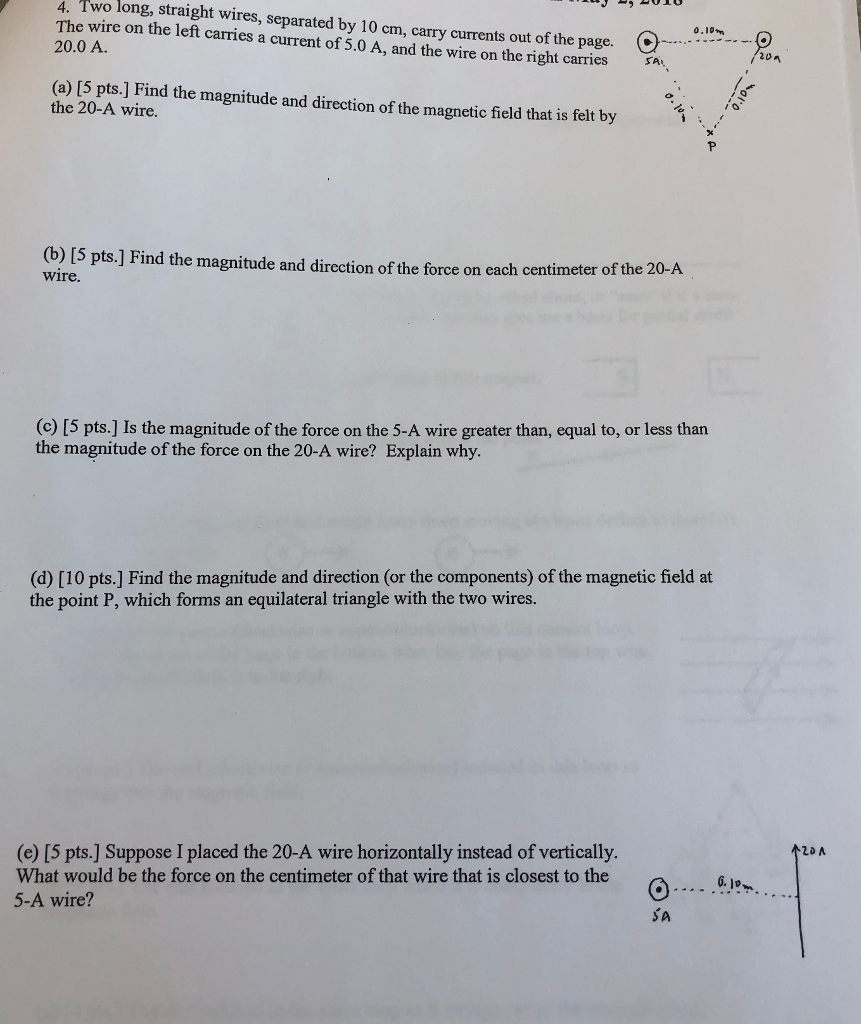4. Two long, straight wires, separated by 10 cm, carry currents out of the page. The wire on the left carries a current of 5.0 A, and the wire on the right carries 20.0 A. 0.10 C 20 (a) [5 pts.] Find the magnitude and direction of the magnetic field that is felt by the 20-A wire. (b) [5 pts.] wire. Find the magnitude and direction of the force on cach centimeter of the 20-A (c) [5 pts.] Is the...

• ### The figure below shows two long, parallel wires incross-section, and the currents they carry are...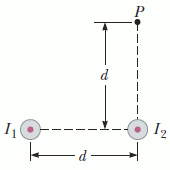The figure below shows two long, parallel wires in cross-section, and the currents they carry are directed out of the page. The left wire carries a current ofI1 = 2.96 A,and the right carries a current ofI2 = 3.45 A.The two wires are a distanced = 20.0 cmapart. Point P is the same distance d above the right wire.Assume the +x-direction is to the right, and the +y-direction is upward.A cross-sectional end view of two parallel wires carrying current out...

• ### PLEASE HELP!!! 8. Two long, parallel wires each carry a current I in the same direction...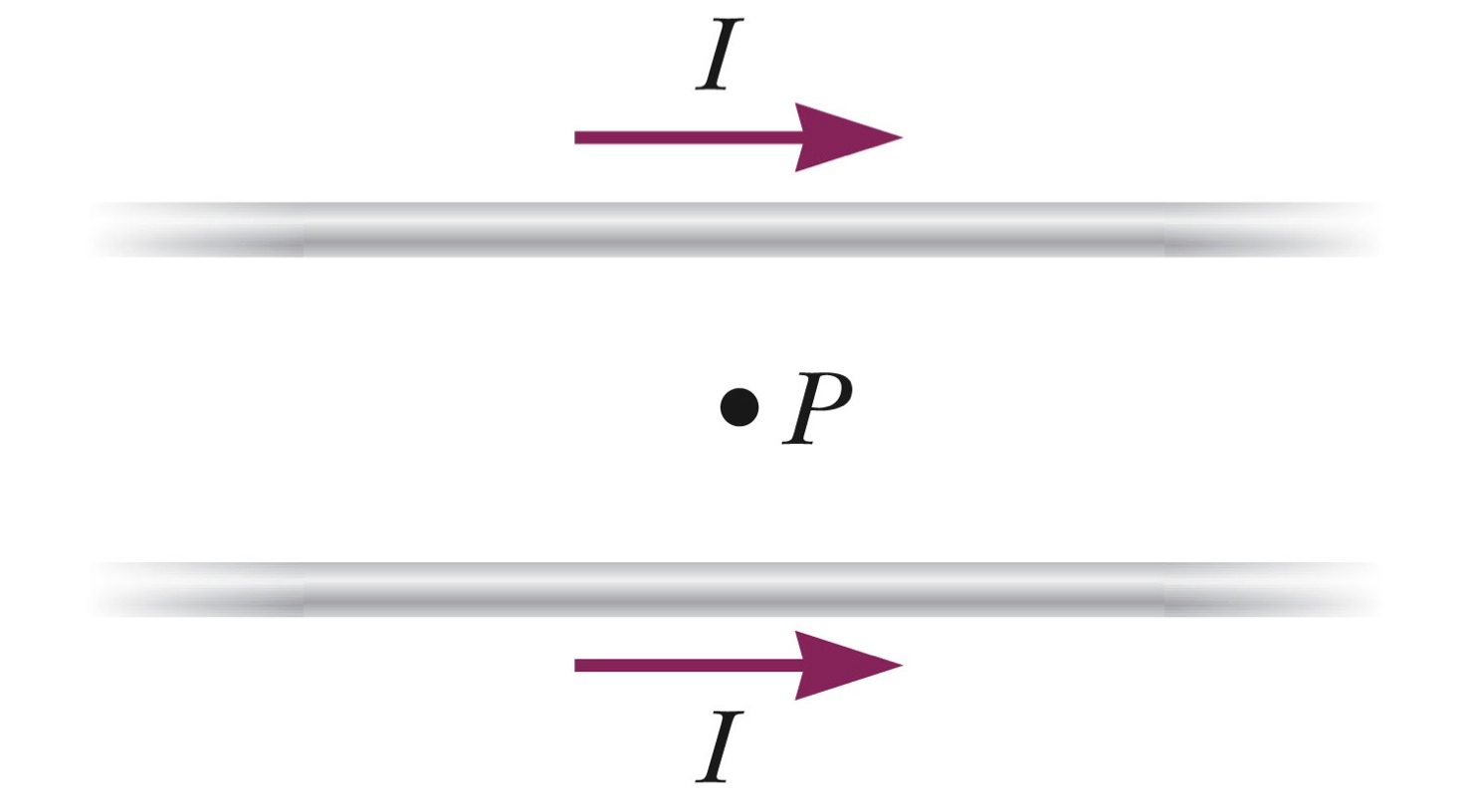PLEASE HELP!!! 8. Two long, parallel wires each carry a current I in the same direction as shown in the figure. The magnetic field at the point P, midway between the two wires is ________. zero directed into the page directed out of the page parallel to the wires in the same direction as the current. parallel to the wires, in the opposite direction as the current. 9. A long, straight wire carries a current of 20A to the right....